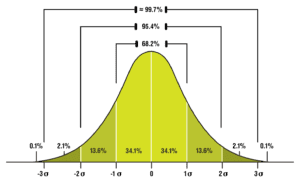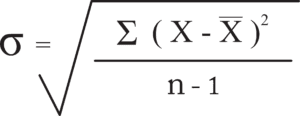# Standard Deviation: What is Six Sigma?

Six Sigma is a data-driven quality control method whose name is derived from the statistical operation known as standard deviation.

Standard deviation is, in short, a measure of spread or variance.

It can be thought of as the average distance individual data points are from the data set’s average.

Standard deviation and sigma, the eighteenth letter of the Greek alphabet, are not always exciting topics to discuss.

This brief exploration aims to keep it short and sweet while retaining the essence of statistical variance and the core concept of the Six Sigma program.

Standard deviation is the key to understanding the term “Six Sigma,” but before asking “What does Six Sigma mean?” we should explore the nature of the program.

## Six Sigma Quality

Six Sigma is, at its heart, a quality control program. That means reducing defective production to the smallest possible frequency of occurrence.

Because Six Sigma originated within the manufacturing sector, it is common to refer to defects as manufacturing defects, but the reality is that a wide variety of processes and systems can benefit from a commitment to Six Sigma quality.

The aim of a Six Sigma program is threefold and is based on three key assumptions.### Stability and Predictability

This foundational assumption is the key to success with Six Sigma.

It focuses team efforts and creates a culture of quality and improvement around the concept that the elimination of variation is a vital business function.

In one way or another, this initial goal informs the rest of the Six Sigma program. This guiding principle pairs very well with the kaizen aspect of the Lean manufacturing system. It is no surprise, then, that the hybrid framework of Lean Six Sigma was born to play off the strengths of each methodology.

### Measured, Analyzed, Improved, and Controlled

This aspect of Six Sigma is codified in the program’s core doctrine of DMAIC – Define, Measure, Analyze, Improve, and Control. Like Kaizen within Lean, DMAIC is a driver of innovation and improvement.

The premise here is that if we quantify a process, we can improve it and apply that same quantification to how much we have improved it.

Six Sigma is built around statistics and statistical tools.

It relies largely on the collection of data and the trust placed in data. There are many kinds of data, but the bottom line here is that the DMAIC process is the guide to the ways in which Six Sigma practitioners bring about the stability and predictability (read: reduction in variance) that is the focus of this program.

### Organizational Commitment

This is huge.

No matter how robust an organization’s suite of statistical tools or how accurate their data collection, a failure to commit to the program will always produce half measures of success.

This is part of the recent conversation surrounding people versus process within the production community. See this article for more (Chief Executive), or join the community conversation here on Quora.

An investment in people only can overcome processes that are obstacles, but an investment in process alone will be hampered by a talent gap. That middle ground is the aim of many decision makers, but an organization can’t even progress to that point without a total commitment to the program.

## The Letter Sigma

As we saw in the foundational pillars of Six Sigma, the program is designed to do the following:

• Improve business processes in predictable and stable ways
• Rely on statistical control to Define, Measure, Analyze, Improve, and Control
• And do so through total organizational commitment

Now let’s look at sigma and standard deviation, the two components of the program’s name.

The term “sigma” has a few relevant definitions.

First and foremost, sigma is a letter. It is the eighteenth letter of the Greek alphabet. Written in its uppercase form it is the summation mathematical operator (Σ).

Summation is the addition of a sequence of numbers.

Written in its lowercase form it is the mathematical symbol for standard deviation (σ).

The standard deviation form is by far the more interesting aspect of sigma, and it ties the term to the Six Sigma (or 6σ) methodology.

## Standard Deviation (the short version)

Standard deviation is the average distribution of variation within a data set. In other words, it’s how spread out individual data points are.

To find the average (or mean) of a set of numbers, we take the sum of those numbers and divide it by the number of values within the data set.

Standard deviation seeks to find variance in the form of the average distance from the arithmetical mean (calculated average).

In the instance of Six Sigma, standard deviation relates to data that can be expressed as fitting a normal distribution. A normal distribution curve, sometimes known as a “bell curve,” is a plot of data where the three key measures of central tendency are all in the graph’s center.

These three key measures of central tendency are as follows:

Mean – Arithmetical mean or calculated average

Median – The “middle value” or the value that falls in the center of the data when it is ordered

Mode – The most commonly occurring value

In the image below, we can see normal distribution in a classic bell curve. As we would expect with the measures of central tendency clustered around the center, the graph spikes in the middle, then tapers off in either direction.Using the formula for standard deviation (below) we can calculate a standard deviation value. With this value, we can move along the normal distribution curve in either the positive or negative direction by a unit the size of a single standard deviation (1σ).Referring to our normal distribution curve, the shaded area under the curve represents 100% of the data. If we are at the zero point (the center of the curve) a large portion of the data points will be concentrated there.

As we move along the curve in either direction, our scope includes a larger portion of the area under the curve, and therefore, a larger portion of the data points. The very far ends of the curve represent outliers, or data points that are anomalous or infrequent.

## 6σ

The program’s name comes from the area under the normal distribution curve, after moving six standard deviations away from the center (three σ in each direction).

Because data that can be expressed as a normal distribution curve tends to behave in specific ways, we can calculate exactly how much of the data is included in the area under the curve at each sigma interval.Moving one standard deviation away from zero (the graph’s center) covers about 34.1% of the data. Moving one standard deviation away from zero in each direction (2σ) therefore covers twice as much, or 68.2% of the data.

Two standard deviations in either direction (4σ) covers 95.4% of the data.

Three standard deviations in either direction (6σ) covers roughly 99.7% of the data.

The goal here is to achieve what is commonly known as “Six Sigma Quality” or defect rates that fall outside the 6σ range. These defect rates are measured in the units DPMO, or defects per million opportunities.

In other words, approximately 99.7% of the data points will consist of output with zero defects.

## The Bottom Line

The bottom line is that Six Sigma so heavily relies on statistical tools and methods that even its name is a product of the world of statistics.

This exploration of the topic of Six Sigma and standard deviation is by no means an in-depth look; the topic is a broad and complex one.

The key takeaway here is to understand just how deep an influence statistical tools and methods have on the Six Sigma program, along with the foundational aspects of the framework.

### The next steps to take:

1. If you found this post helpful, take a moment to share it. Even better, tell us in the comments!
2. Continue exploring the world of Lean thinking and process optimization with these helpful posts:

Learn to spot, classify, and eliminate waste.

Simplicity in practice: the 5S system

Calculate standard deviation.

• Email
• More Networks
[class^="wpforms-"]
[class^="wpforms-"]
[class^="wpforms-"]
[class^="wpforms-"]
[class^="wpforms-"]
[class^="wpforms-"]
[class^="wpforms-"]
[class^="wpforms-"]
[class^="wpforms-"]
[class^="wpforms-"]
[class^="wpforms-"]
[class^="wpforms-"]
[class^="wpforms-"]
[class^="wpforms-"]
[class^="wpforms-"]
[class^="wpforms-"]
[class^="wpforms-"]
[class^="wpforms-"]
[class^="wpforms-"]
[class^="wpforms-"]
[523.251,1046.50]
[523.251,1046.50]
[523.251,1046.50]
[523.251,1046.50]
[523.251,1046.50]
[523.251,1046.50]
Share via